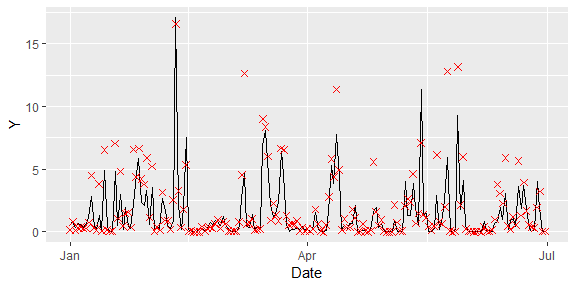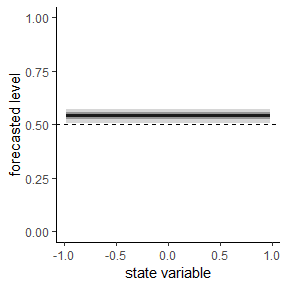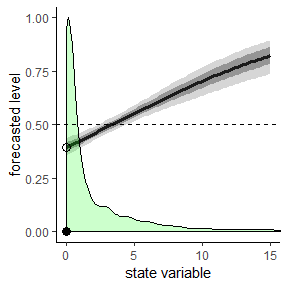Data


library(PointFore)
library(ggplot2)
library(lubridate)
#>
#> Attaching package: 'lubridate'
#> The following object is masked from 'package:base':
#>
#>     date

precipitation$Date <- as.Date(row.names(precipitation),format = "%d-%m-%Y") ggplot(subset(precipitation, month(Date)< 7 & year(Date)==2013))+ geom_line(aes(x=Date,y=Y))+ geom_point(aes(x=Date,y=X), color = 'red', size = 2, shape=4)Time series of one-day ahead HRES Precipitation forecasts (crosses) and respective observations (solid line) over London. For more information on the data see ?precipitation. Analysis Now, let us analyse the forecasts. We begin with the constant expectile model.  instruments <- c("lag(lag(Y))","X") res <- estimate.functional(iden.fct = expectiles, model = constant, instruments = instruments, Y = precipitation$Y, X=precipitation$X) #> Drop 2 case(s) because of chosen instruments #> Choose parameter theta0 automatically. summary(res) #>$call
#> estimate.functional(iden.fct = expectiles, model = constant,
#>     Y = precipitation$Y, X = precipitation$X, instruments = instruments)
#>
#> $coefficients #> Estimate Std. Error t value Pr(>|t|) #> Theta 0.5419114 0.020512 26.41924 8.237429e-154 #> #>$Jtest
#>
#>  ##  J-Test: degrees of freedom is 2  ##
#>
#>                 J-test      P-value
#> Test E(g)=0:    3.9107e+01  3.2220e-09
plot(res,hline = TRUE)Constant expectile analysis.

Optimality is rejected with a p-value of 0. On average the forecast tends to overestimation compared to an optimal mean forecast with an expectile level of 0.54.

Next, we consider state-dependent forecasting behavior. Instead of using the conventional state-dependence models we rely on the linear probit specification model but enforce an expectile level of $$0$$ for the forecast $$0$$. This is a logical consequence of precipitation being a positive random variable.

probit0 <- function(stateVariable,theta) probit_linear(stateVariable, theta)*(stateVariable>0)

res <- estimate.functional(iden.fct =   expectiles ,
model = probit0,
theta0 = c(0,0),
instruments = instruments,
state = precipitation$X, Y = precipitation$Y, X=precipitation$X) #> Drop 2 case(s) because of chosen instruments summary(res) #>$call
#> estimate.functional(iden.fct = expectiles, model = probit0, theta0 = c(0,
#>     0), Y = precipitation$Y, X = precipitation$X, stateVariable = precipitation$X, #> instruments = instruments) #> #>$coefficients
#>             Estimate Std. Error   t value     Pr(>|t|)
#> Theta -0.26720297 0.06458326 -4.137341 3.513543e-05
#> Theta  0.08056457 0.01530689  5.263287 1.415021e-07
#>
#> \$Jtest
#>
#>  ##  J-Test: degrees of freedom is 1  ##
#>
#>                 J-test   P-value
#> Test E(g)=0:    0.11280  0.73698

To replicate the result plot in the paper , we need to adjust the standard plot function of the PointFore package to the probit0 specification model.

plot(res,limits = c(0.001,15),hline = TRUE)+
geom_point(data=data.frame(x=c(0,0),y=c(0,.395),shape=c(1,2)),
aes(x=x,y=y,shape=as.factor(shape)),
,size=3,show.legend = FALSE)+
scale_shape_manual(values=c(16,1))Plot linear probit model.